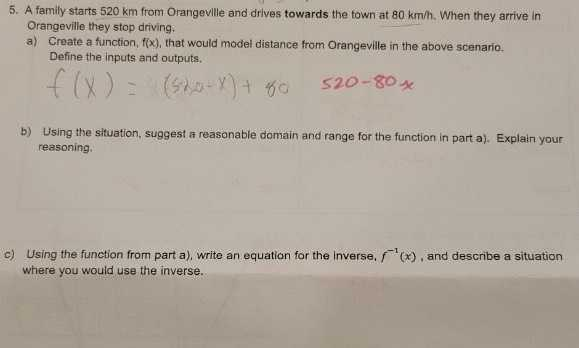### ¿Todavía tienes preguntas de matemáticas?

Pregunte a nuestros tutores expertos
Algebra
PreguntaA familystarts $$520 km$$ from Orangeville and drives towards the town at $$80 km / h$$ . When they arrive in Orangeville they stop driving.

a) Create a function, $$f ( x )$$ , that would model distance from Orangeville in the above scenario. Define the inputs and outputs,

b) Using the situation, suggest ci reasonable domain and range for the function in part a). Explain your reasoning.

c). Using the function from part a), write an equation for the inverse, $$f ^ { - 1 } ( x )$$ , and describe a situation where you would use the inverse.

$$a)f(x)= 520- 80x,$$input : times;output :distance from Orangeville and drives.
c)$$f^{- 1}(x)= 6.5- \frac{1}{80}x$$